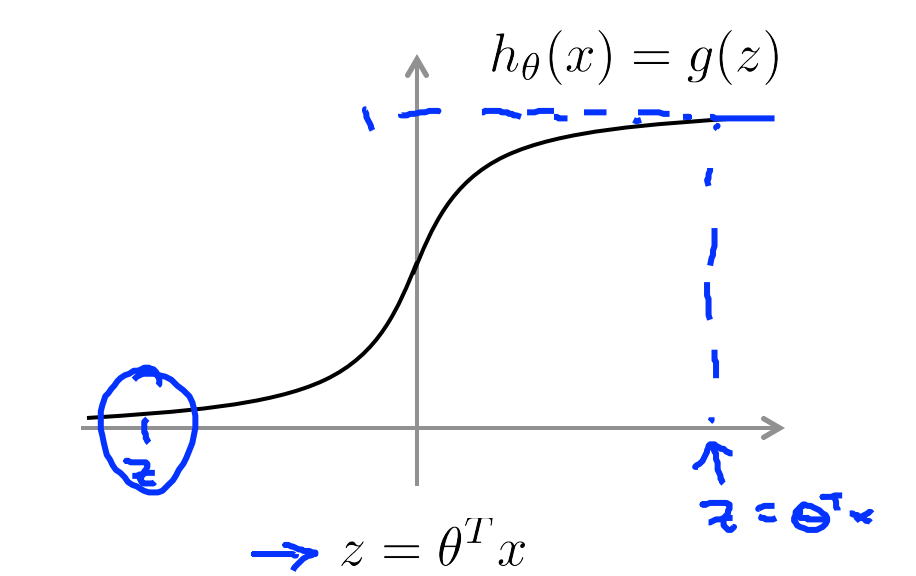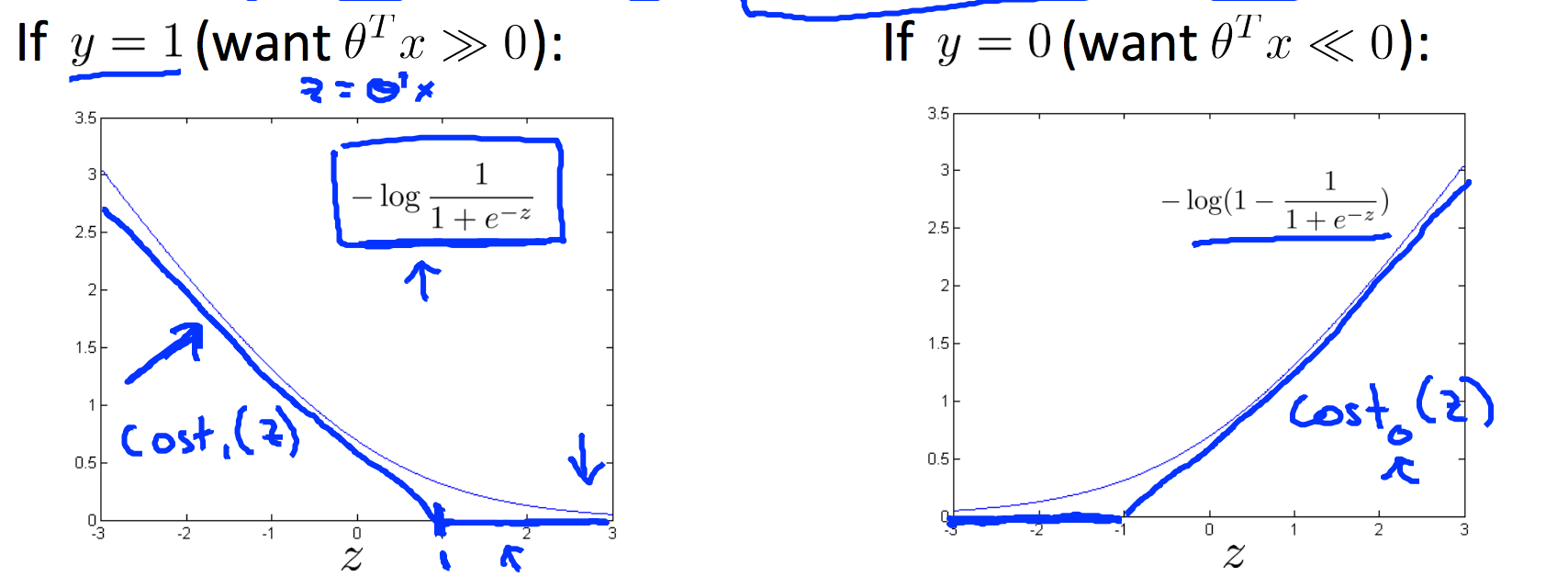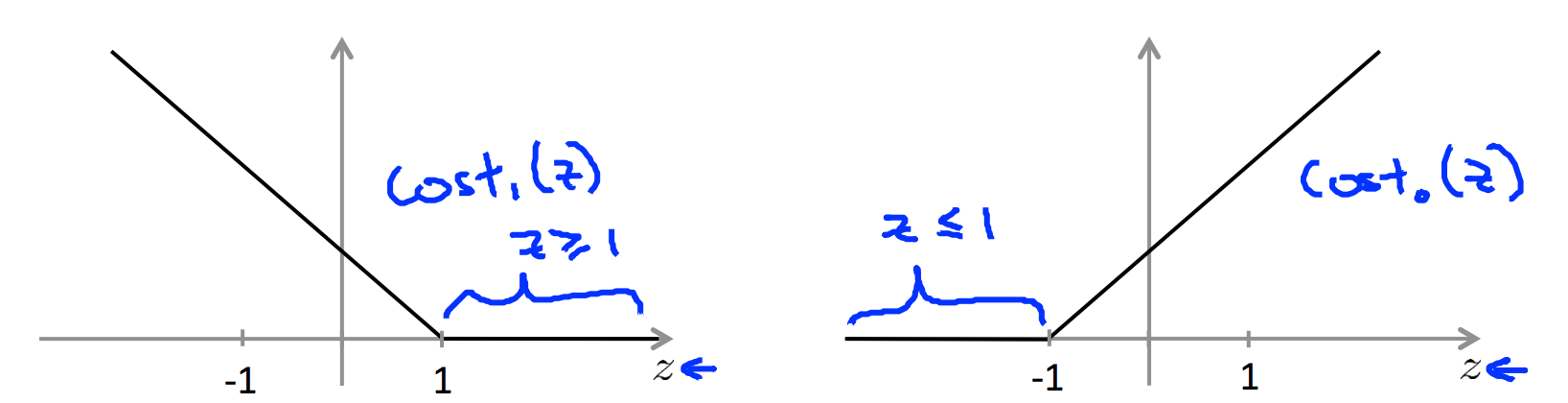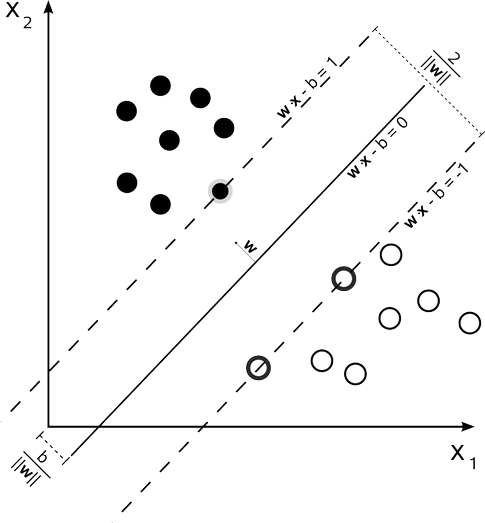# SVM

• SVM定义
• 最大间隔分类器-Large Margin Classification
• 核函数-Kernels
• SVM操作实例-SVMs in Practice

## SVM定义

SVM 可以进行线性和非线性的分类：

### 线性分类

: 给定一组训练实例，每个训练实例被标记为属于两个类别中的一个或者另一个，SVM训练算法创建一个新的实例分配给两个类别之一的模型，使其成为非概率的二元线性分类器。SVM将实例表示为空间中的点，这样映射就使得单独类别的实例被尽可能宽的明显分隔开，然后，将新的实例映射到同一空间，并基于它们落在间隔的哪一侧来预测

### 非线性分类

: 除了线性分类之外，SVM还可以用核技巧有效的进行非线性分类，将其输入隐式映射到高维特征空间中。

## 最大间隔分类器（Large Margin Classification）

由logistic回归出发，引出SVM，既揭示了模型间的联系，也让过渡更加自然。


### 优化目标函数（Optimization objective）

#### 逻辑回归的回顾

${h}_{\theta }\left(x\right)=\frac{1}{1+{e}^{-{\theta }^{T}x}}$$-\left(y\mathrm{log}{h}_{\theta }\left(x\right)+\left(1-y\right)\mathrm{log}\left(1-{h}_{\theta }\left(x\right)\right)\right)\phantom{\rule{0ex}{0ex}}=-y\mathrm{log}\frac{1}{1+{e}^{-{\theta }^{T}x}}-\left(1-y\right)\mathrm{log}\left(1-\frac{1}{1+{e}^{-{\theta }^{T}x}}\right)$

#### 支持向量机

$\underset{\theta }{min}\frac{1}{m}\left[\sum _{i=1}^{m}{y}^{\left(i\right)}\left(-\mathrm{log}{h}_{\theta }\left({x}^{\left(i\right)}\right)\right)+\left(1-{y}^{\left(i\right)}\right)\left(\left(-\mathrm{log}\left(1-{h}_{\theta }\left({x}^{\left(i\right)}\right)\right)\right)\right]+\frac{\lambda }{2m}\sum _{j=1}^{n}{\theta }_{j}^{2}$

$\underset{\theta }{min}C\sum _{i=1}^{m}\left[{y}^{\left(i\right)}\left(cos{t}_{1}\left({\theta }^{T}{x}^{\left(i\right)}\right)+\left(1-{y}^{\left(i\right)}\right)\left(cos{t}_{0}\left({\theta }^{T}{x}^{\left(i\right)}\right)\right]+\frac{1}{2}\sum _{j=1}^{n}{\theta }_{j}^{2}\phantom{\rule{0ex}{0ex}}s.t.C=\frac{1}{\lambda }$### 最大间隔解释（Large Margin Intuition）

$\underset{\theta }{min}C\sum _{i=1}^{m}\left[{y}^{\left(i\right)}\left(cos{t}_{1}\left({\theta }^{T}{x}^{\left(i\right)}\right)+\left(1-{y}^{\left(i\right)}\right)\left(cos{t}_{0}\left({\theta }^{T}{x}^{\left(i\right)}\right)\right]+\frac{1}{2}\sum _{j=1}^{n}{\theta }_{j}^{2}$#### SVM 的决策边界（Decision Boundary)

$\underset{\theta }{min}C\sum _{i=1}^{m}\left[{y}^{\left(i\right)}\left(cos{t}_{1}\left({\theta }^{T}{x}^{\left(i\right)}\right)+\left(1-{y}^{\left(i\right)}\right)\left(cos{t}_{0}\left({\theta }^{T}{x}^{\left(i\right)}\right)\right]+\frac{1}{2}\sum _{j=1}^{n}{\theta }_{j}^{2}$

Whenever${y}^{\left(i\right)}=1$$y^{(i)}=1$:

${\theta }^{T}{x}^{\left(i\right)}\ge 1$

Whenever ${y}^{\left(i\right)}=0$$y^{(i)}=0$:
${\theta }^{T}{x}^{\left(i\right)}\le -1$

$\underset{\theta }{min}\frac{1}{2}\sum _{j=1}^{n}{\theta }_{j}^{2}\phantom{\rule{0ex}{0ex}}s.t.{\theta }^{T}{x}^{\left(i\right)}\ge 1\phantom{\rule{1em}{0ex}}if{y}^{\left(i\right)}=1\phantom{\rule{0ex}{0ex}}{\theta }^{T}{x}^{\left(i\right)}\le -1\phantom{\rule{1em}{0ex}}if{y}^{\left(i\right)}=0$

SVM目的是最大化间隔（支持向量和决策边界之间的间隔）

## 形成式表示${h}_{w,b}\left(x\right)=g\left({w}^{T}x+b\right)$

## 支持向量机的数学表达

#### 函数间隔（functional margin）和几何间隔（geometric magin）

• 1.对于任意一条给定的直线（面）即超平面，找到所有距离这条直线最近的样本点（可能有一个，可能有多个）

• 2.计算这些样本点到直线的距离，求出所有可能的最大值

1. 首先定义直线（超平面）：
$y\left(x\right)={w}^{T}x+b$
2. 任意一点到直线的距离为：
$\frac{1}{‖w‖}\left({w}^{T}x+b\right)$

其中，$‖w‖$$\Vert w\Vert$是w的范数，几何意义上表示一个向量的长度
3. 根据上述的最大间距的描述，我们使用公式：
$\underset{w,b}{max}\left\{\frac{1}{‖w‖}mi{n}_{n}\left[{y}^{i}\left({w}^{T}x+b\right)\right]\right\}$

该公式精确的上述对支持向量机的描述的理解：先找出所有可能的直线、平面，满足条件就是样本点到该直线的最小距离。这个集合中的每个元素至少包含两个信息：（1）距离最近超平面最近的点（2）点到直线或者平面的距离。然后再在这个集合中找到间距最大的那一个，就是我们所求的最优解。
但是该公式还是比较复杂，所以我们提出函数间隔的定义：

#### 函数间隔

${\stackrel{^}{r}}^{\left(i\right)}={y}^{\left(i\right)}\left({w}^{T}{x}^{\left(i\right)}+b\right)$

&#8194 继续考虑w和b，如果同时加大w和b，那么所有的函数间隔都会增大，但是事实上该问题不应该影响结果。所以，为了解决这个问题，我们可能需要加入归一化条件，毕竟求解的目标是确定唯一一个w和b，而不是多组线性相关的向量。这个归一化等一会再考虑。

$\stackrel{^}{r}=\underset{i=1,2,...,m}{min}{\stackrel{^}{r}}^{\left(i\right)}$

### 目录